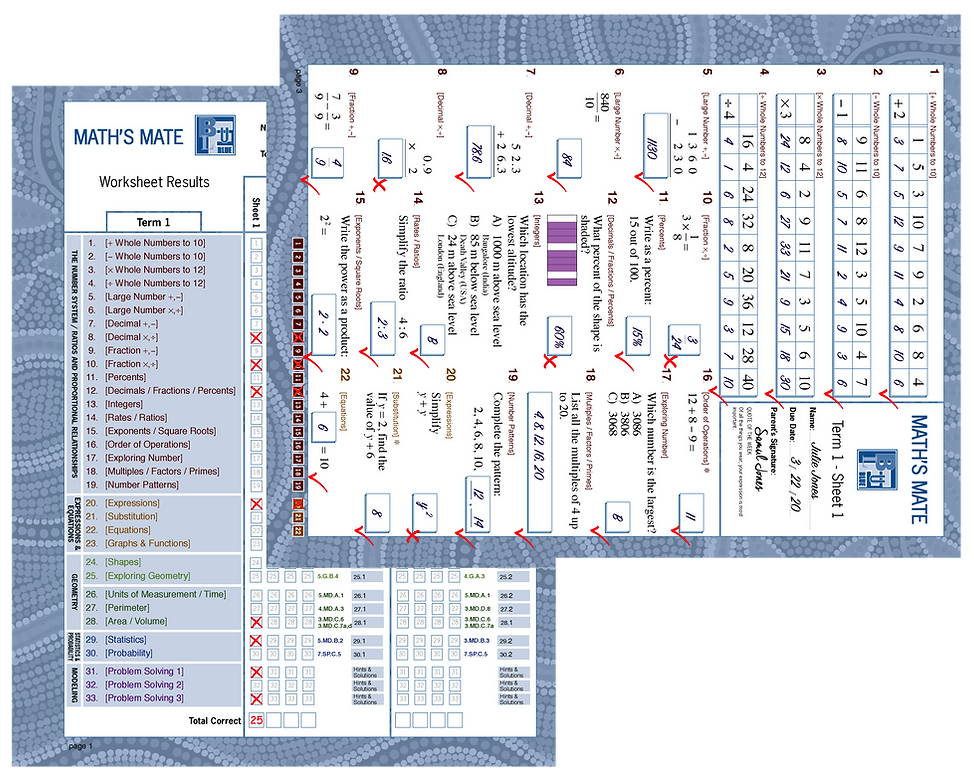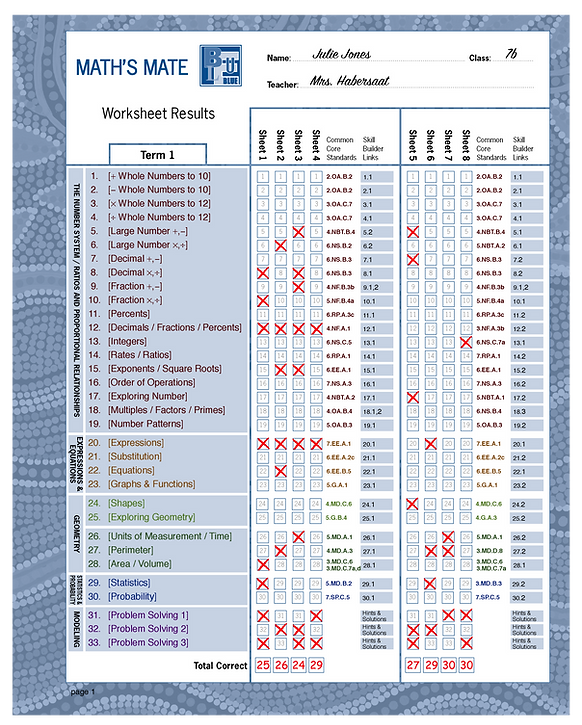# How Math's Mate Works

The Math's Mate Program is based on the principle that regular, structured practice helps the development of short and long term memory.  Through systematic weekly revision, a student's mathematical knowledge, skills and ability to solve problems are consolidated and extended.

Each question on a Math's Mate worksheets is part of a set of 4 similar questions in the term.

For example, consider sheets 1, 2, 3 and 4 in Math's Mate Blue term 1. Question 12 on each sheet is similar in design, content and degree of difficulty. This grouping of question style is also true of the next set of four sheets and so on.

The Math's Mate results worksheet found at the start of each term in every Student Workbook, enables students to find out what they know and what they still need to learn and practice.

How to Use the Program

##### 1. Complete the Worksheet

Student fills out the Math's Mate Worksheet. Parent signs the work.##### 2. Correct Completed Work

Students correct their work in class.

Over successive weeks strengths and weaknesses in particular skills become evident.

##### 3. Test Students

The Teacher Resources includes A and B tests that can be completed after every four worksheets. These tests ensure diagnostics are accurate.##### for Easy Reporting

The Math's Mate results sheet provides teachers with a record of each student's progress allowing them to clearly show parents their child's strengths and weaknesses.The appropriate Skill Builders can be assigned as identified on the record sheet.

##### 6. Completes Skill Builder

Students are supported with instructions, worked examples, Glossary, Math Facts, and further exercises to complete.##### 7 Correct Skill Builders

Teacher encourages student's efforts to get on top of all key skills.

### The MATH'S MATE BUNDLE

When schools purchase a class set of Math's Mate workbooks the teachers are supported with free Teacher Resources and Skill Builders. These make the teacher's job as simple as possible, and the cost of running the program very reasonable. Contact us for more information.

## SKILL BASED LEARNING

All skills acquired by students at each Math's Mate level relate to key mathematical strands.  Every strand is revised weekly.

## SYSTEMATIC STRUCTURE

Math's Mate has a consistent structure across all levels. Each term progressively increases in difficulty as the student strengthens their skills. The advanced (White) level is an in-between level for students that need an extra challenge. The blue shaded area on the graph shows the typical range of difficulty for each of the student workbooks.

## Skill Builders

Math's Mate provides four Skill Builder editions, each spanning two year levels (Orange/Rose, Yellow/Red, Blue/Green and Mauve/Lime) to support quick, easy and helpful remediation for students who have difficulty in any particular mathematical area.

Each Skill Builder consists of:

• Step-by-step instructions on how to solve a typical question

• A worked example

• Practice questions

• A Glossary of important terms

• Math Facts

How to use Skill Builders
1. Determine which Math's Mate questions pose a difficulty
2. Find the relevant Skill Builder on the Math's Mate results worksheet
3. Look up any unknown terms in the Skill Builder glossary
4. Complete the relevant Skill Builders
5. Correct the Skill Builders from the Skill Builder answer sheets
6. Circle the completed skill numbers on the Maths Mate results worksheet
7. Go back and repeat previous Math's Mate questions

Maths Mate design and how Skill Builders relate

Any question on the Math's Mate worksheets is part of a set of 4 similar questions in the term.
For example, consider sheets 1, 2, 3 and 4 in Math's Mate Blue term 1.
Question 12 on each sheet is similar in design, content and degree of difficulty.
This grouping of question style is also true of the next set of four sheets and so on.  Thus the Math's Mate tests made available in the Teacher Resource PDF, also reflect this grouping of question style and substance.

The Math's Mate worksheet results sheets found at the start of each term in each Student Pad (and in each Teacher Resource PDF) enable students to find out what they know and what they still need to learn and practice.

The relevant Skill Builder numbers are listed at the end of each set of 4 questions on the record keeping sheets.

Linking Skill Builders back to Math's Mate

Similarly too the Skill Builders can be linked back to each set of 4 similar Math's Mate questions.  These links are identified in the grid at the title of each skill.

(See e.g. 13.3 below from Skill Builder Blue/Green)

The grid shown here for example, would relate a skill to question 19 in: the last 4 sheets of MM Blue term 2,
and the last 4 sheets of MM Green term 1.

Once understood these links will be helpful to students, in their selection of Skill Builders, and teachers, in their allocation of Skill Builders to students.